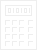EarlyElectronicCalculator Calculator SimulationsHere are simulations of desktop calculators from the late 1960s. These simulations show how the calculators operate internally, from handling numeral entries to the details of the multiplication and division algorithms.

These simulations were created after completing level 2 (logic schematic) reverse engineerings of the calculators to assist in moving to level 3. (For an explanation of the levels see the comments on reverse engineering.) The first one, for the Facit 1123, started out as an assistance to repairing the unit.

Calculator Simulation Simulation Type
Facit 1123 Run Logic
Toshiba BC-1212 Run Algorithmic
Sharp Compet 17 Run Logic
Monroe 925 Run Logic
Casio AL-1000 Run Logic
Casio 121-B Run Logic

 Requirement: The simulations are ShockWave programs and require the MacroMedia ShockWave plug-in for your browser. Suggestion: Running a simulation opens a new window in your browser. When finished with the simulation, close the window to return here. The simulations require a lot of screen real estate, you may be able to improve the display by minimizing browser tool bars and such. In Netscape, click on the dotted bars at the left of the tool bars. Suggestion: The BC-1212 simulation is faster and the algorithms easier to comprehend than the others.

These calculators are representative of electronic calculators sold to the business and accounting communities in the late 1960's.

Calculators of this time (just before the advent of microprocessors) and market were typically fully serial machines (one-bit wide arithmetic unit and data paths), processing numbers in BCD (Binary Coded Decimal) or 1242 format, controlled by a hard-wired state machine.

The bit-at-a-time serial design harkens back to some of the first digital electronic computers of the late 1940s and early 1950s, such as the SSEM, but implemented in the new solid-state integrated circuit technology of the 1960s. Bit-serial processing minimizes the amount of hardware needed and consequently the cost and complexity, at the expense of speed. For the first computers the reduced speed was undesirable, for manually-operated calculators it was irrelevant.

Two approaches were taken to producing these simulations:

• Logic Simulation:
At the heart of the simulation is a logic simulation engine. A complete description of the gates and connections of the calculator, based on the logic schematic, is supplied to the engine. The engine constructs a network of simulated gates (over 3000 objects for the 1123) to represent the calculator.

The engine then accepts external events (key presses and clock pulses) and sends them into the network where they propagate about until eventually resulting in changes to the relevant displays and events sent back to the engine.

• Algorithmic Simulation:
A slightly higher-level simulation which implements the sequencing and algorithms of the original in code. The logic schematic is manually examined to determine it's operation and then "re-phrased" in code. This approach was taken simply because the logic design of the 1212 was elegant enough to make it's interpretation reasonably straightforward.

The control (state machine) portion of the calculator becomes primarily a bunch of boolean expressions. The data and arithmetic portions are simulated at the decimal digit level. That is, the simulation processes one decimal digit at a time. This is a break from the original, which processes one bit at a time, with 4 bits per decimal digit. Making this break was done for several reasons:

• It speeds up the simulation.
• The control section controls the data at the decimal digit level. Bit-level operations below the decimal digit level are inconsequential to the control section.
• Consequently, it is a sufficient level of simulation to expose the principles of the numeral handling and decimal arithmetic algorithms.
Simulations at these levels mean the flaws and anomalies of the originals are also present in the simulations.

One of the fun parts of producing the simulations is adding the optional features which were left out of the original unit. For example, in the 1123 the simulation is based on there is actually no user memory or 5/4 rounding switch. Inside the unit the locations for the extra keys and ICs can be discerned. After the reverse engineering it was simple to add these features to the simulation.

 Calculators | Integrated Circuits | Displays | Simulations EEC Aug 2000